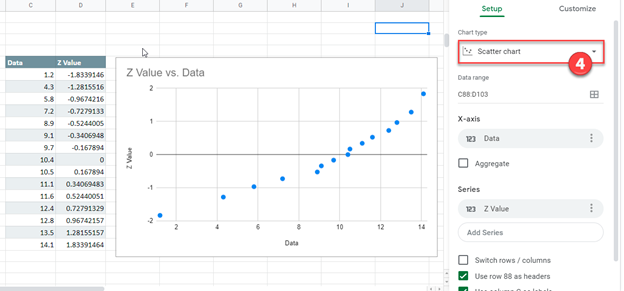# Normal Probability Plot – Excel & Google Sheets

This tutorial demonstrates how to create a Normal Probability Plot in Excel & Google Sheets.

## Normal Probability Plot – Excel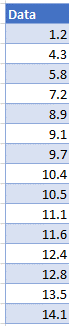### Calculate the Z Values

In the next column, create a formula with the NORM.S.INV, RANK, and COUNT Functions:

``=NORM.S.INV((RANK(D5,\$D\$5:\$D\$19,1)-0.5)/COUNT(D:D))``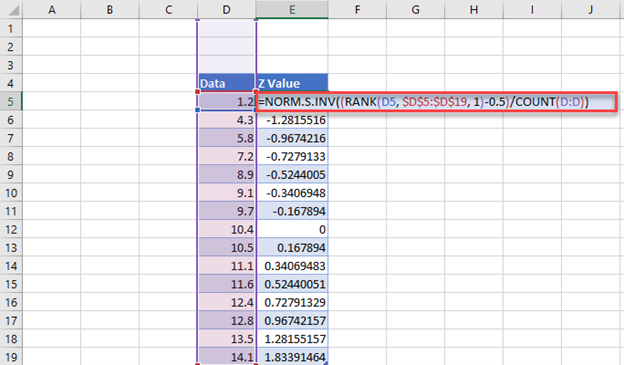### Create Probability Graph

1. Highlight Data
2. Select Insert
3. Click Scatter
4. Select first Scatterplot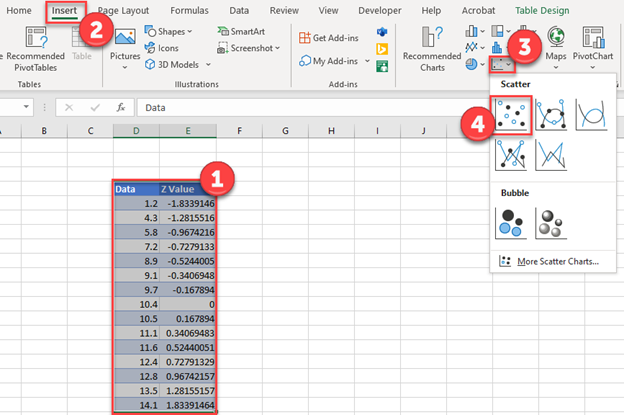### Final Normal Probability Plot

Below, you can see the final normal probability plot.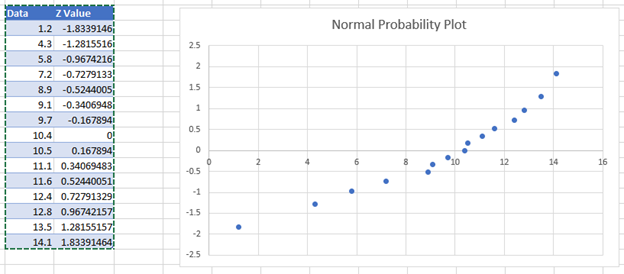### Easy Excel Automation### Normal Probability Plot – Google Sheets

1. Highlight the data
2. Select Insert
3. Click Chart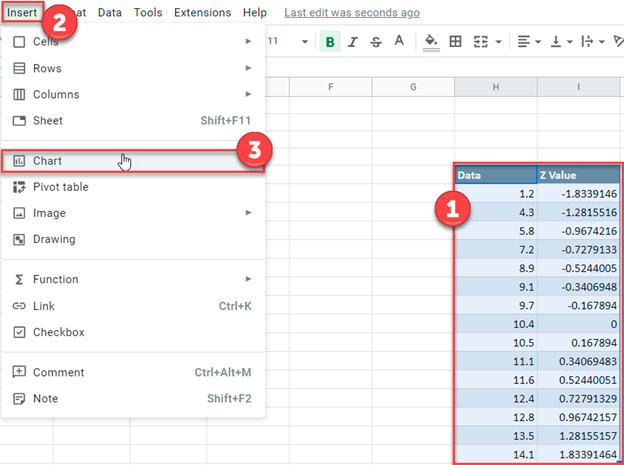4. After changing the Chart Type to Scatter Chart, your graph should look similar to the one below.